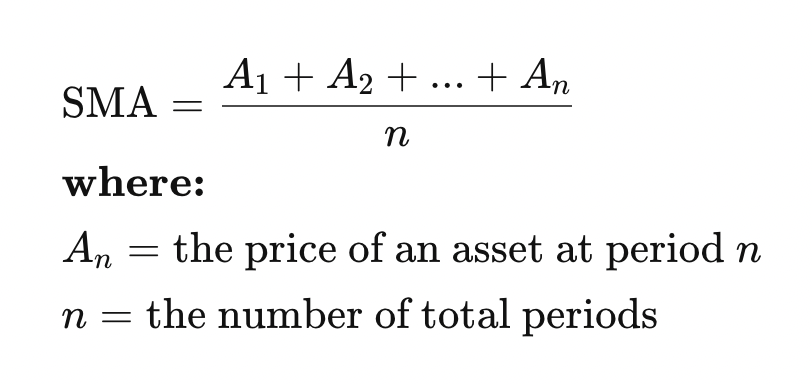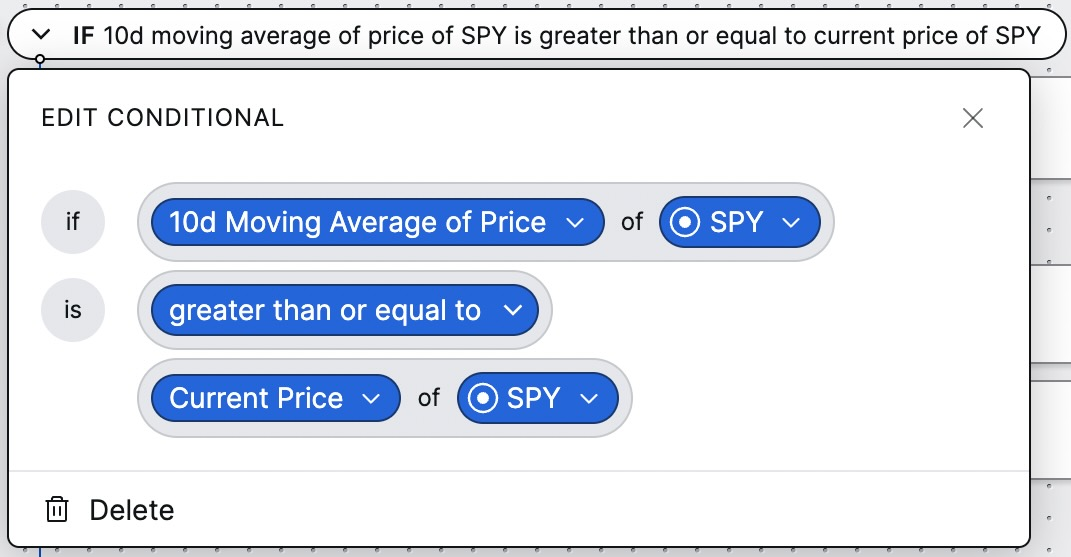# Simple moving average

In this article, you’ll learn about simple moving average of price and how it’s calculated in Composer.

What is Simple Moving Average?

Simple moving average calculates the arithmetic mean price of an asset over a given time period. It can be viewed as a “less-noisy” price of an asset.

Formula

The formula for simple moving average is calculated as follows:Example

For a small-scale example, let’s say we want to calculate the 5-day simple moving average of an asset that’s currently trading at \$10.00 on a Friday. The closing price was \$6.00 on Monday, and it went up a dollar each day, so the closing price for each day looks like this:

• Monday: \$6.00
• Tuesday: \$7.00
• Wednesday: \$8.00
• Thursday: \$9.00
• Friday: \$10.00

While the current price is \$10, the 5-day simple moving average would be \$8.00. This helps smooth out the volatility of an average and is commonly used to indicate uptrends or downtrends.

Where do you see it in Composer?

Simple moving average is used in our Editor tool, most commonly in “If/Else” statements. An example is shown below:In this example, we’re comparing the 10-day simple moving average of SPY to the current price of SPY. In calculation, Composer gathers the closing price of SPY for the last 10 days, sums them, and divides by 10, and compares it to SPY’s current price.

If the 10-day simple moving average of SPY is higher than or equal to the current price, the blocks of the symphony listed below the “If” statement will execute. Otherwise, the blocks listed below the “Else” statement will execute.

What is the step-by-step calculation?

To calculate the simple moving average of an asset:

• Select the asset and time period
• For each day in the time period, log the closing price of the asset. Sum these together and divide the sum by the number of days in the time period
• The output of this will be the simple moving average of the asset